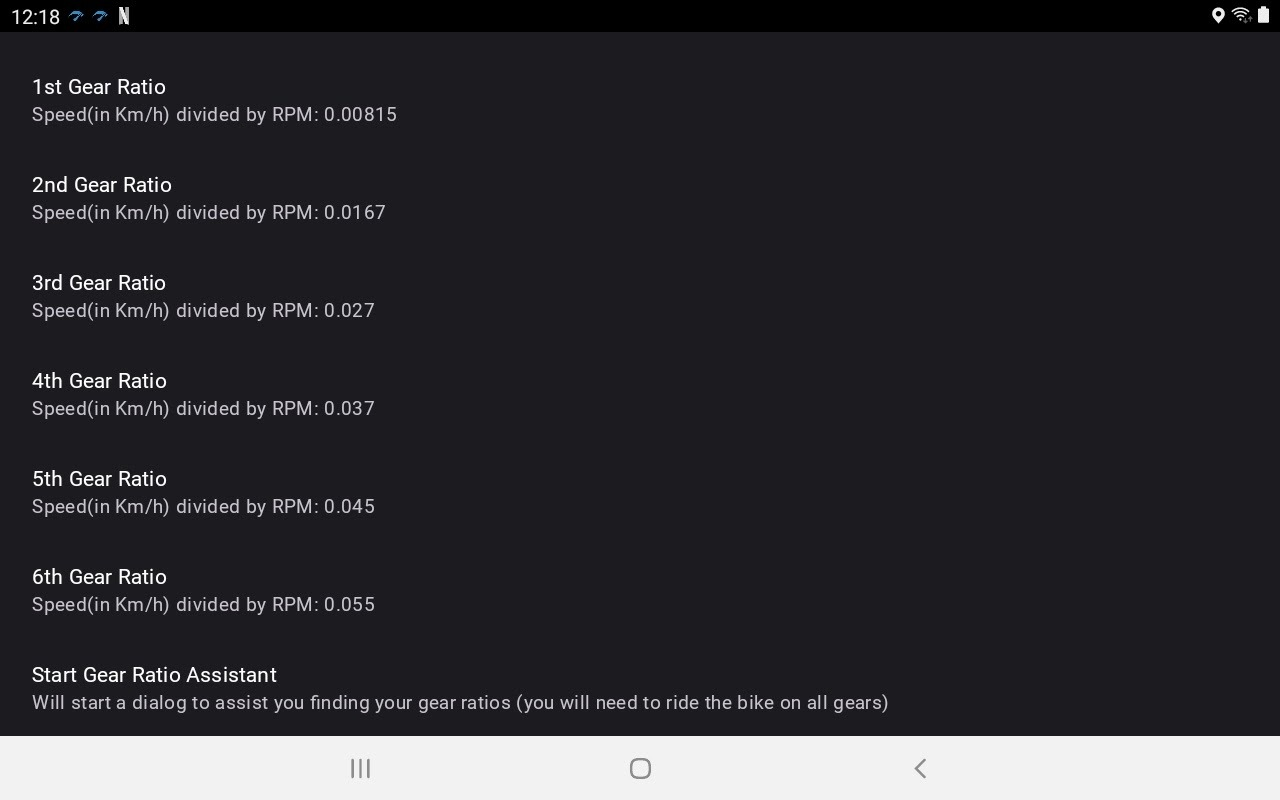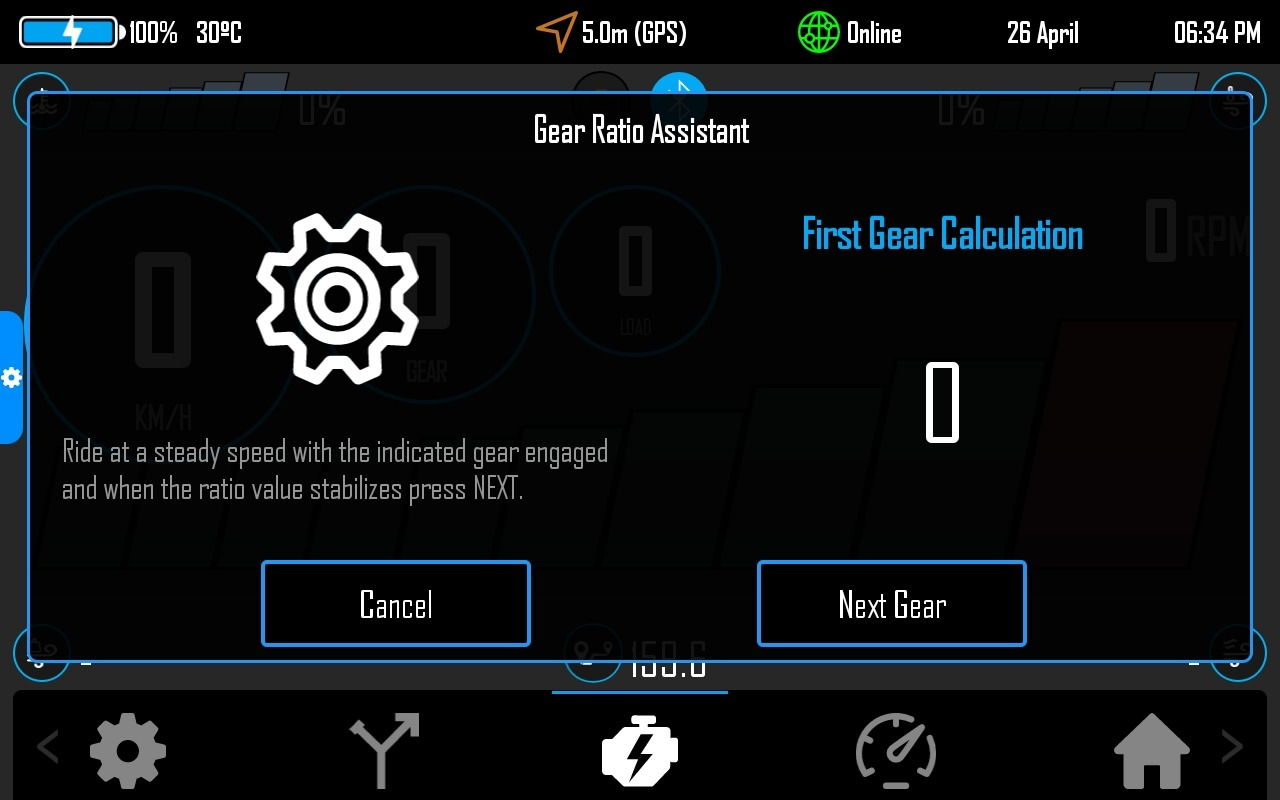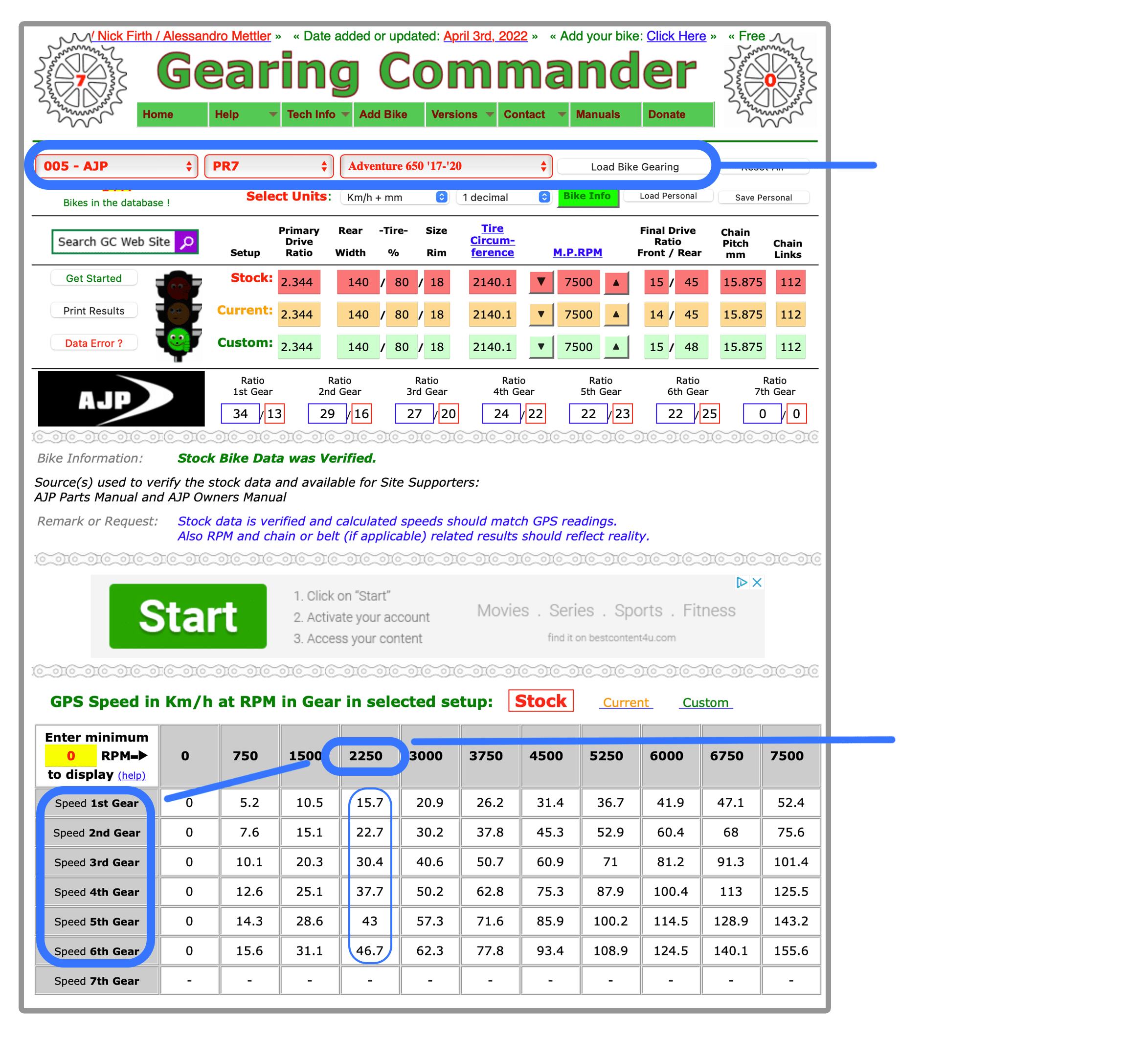# Gear Indicator Setup

For the gear indicator to work you need to configure some values like the number of gears and the ratios for each.

In DMD2 we simply divide SPEED per RPM. So, pull the left menu and go to OBD Setup and look for the Gear Indicator Settings:

You need to specify:

• Number of Gears
• Minimum RPM at which the app will start calculating the gear (should be above Idle RPM)
• The ratio for each gear (by ratio, in our case, its SPEED divided by RPM)# Gear Ratio Calculator Assistant

To make this an easy process we developed our own assistant that allows the app to capture each gear ratio with a single click.

11

Tap here to start the Gear Ratio AssistantIn the OBD view swipe from the left to call the left Menu, then click OBDView Setup.

Scroll down and you will find the Gear Ratio Assistant in the bottom of the options (last option).

# Using Stock Values

To get some initial values you can use the Gearing Commander website, the values should be very close if you are running a stock bike.

1
21

Select your bike and hit “Load Bike Gearing”

2

Now for each gear divide Speed per RPM.

Example for the AJP PR7:

We went to Gearing Commander website and loaded the AJP PR7 values.

Now in the table bellow, we can dow our math for each gear. For this example we are assuming a RPM target above idle, 2250RPM for example (you can use any RPM).

For the first gear:
– We divide 15.7 per 2250, which gives us:
0,0069
(this is the value we input in DMD2 for the first gear)

For the second gear:
– We divide 22.7 per 2250, which gives us:
0,0100
(this is the value we input in DMD2 for the second gear)

And so on for each gear.

Obviously you can get these values very easily and on the road, just ride (preferably coasting) at a comfortable RPM for each gear and check the speed at that RPM, the math is simple, always divide the Speed per RPM at each gear.